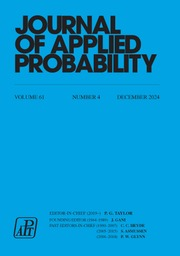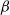Home
Hostname: page-component-5c569c448b-4wdfl Total loading time: 0.289 Render date: 2022-07-04T01:05:07.957Z Has data issue: true Feature Flags: { "shouldUseShareProductTool": true, "shouldUseHypothesis": true, "isUnsiloEnabled": true, "useRatesEcommerce": false, "useNewApi": true } hasContentIssue trueJournal of Applied Probability

## Abstract

It is well known that stationary geometrically ergodic Markov chains are$\beta$ -mixing (absolutely regular) with geometrically decaying mixing coefficients. Furthermore, for initial distributions other than the stationary one, geometric ergodicity implies$\beta$ -mixing under suitable moment assumptions. In this note we show that similar results hold also for subgeometrically ergodic Markov chains. In particular, for both stationary and other initial distributions, subgeometric ergodicity implies$\beta$ -mixing with subgeometrically decaying mixing coefficients. Although this result is simple, it should prove very useful in obtaining rates of mixing in situations where geometric ergodicity cannot be established. To illustrate our results we derive new subgeometric ergodicity and$\beta$ -mixing results for the self-exciting threshold autoregressive model.

## MSC classification

Type
Original Article
Information
Journal of Applied Probability , September 2021 , pp. 594 - 608

## Access options

Get access to the full version of this content by using one of the access options below. (Log in options will check for institutional or personal access. Content may require purchase if you do not have access.)

## References

Bradley, R. C. (1986). Basic properties of strong mixing conditions. In Dependence in Probability and Statistics, eds E. Eberlein and M. S. Taqqu, pp. 165–192. BirkhÄuser, Boston.CrossRefGoogle Scholar
Bradley, R. C. (2005). Basic properties of strong mixing conditions: a survey and some open questions. Prob. Surveys 2, 107144.CrossRefGoogle Scholar
Bradley, R. C. (2007). Introduction to Strong Mixing Conditions, Vols 1–3. Kendrick Press, Heber City.Google Scholar
Chan, K. S., Petruccelli, J. D., Tong, H. and Woolford, S. W. (1985). A multiple-threshold AR(1) model. J. Appl. Prob. 22, 267279.CrossRefGoogle Scholar
Davydov, Y. A. (1973). Mixing conditions for Markov chains. Theory Prob. Appl. 18, 312328.CrossRefGoogle Scholar
Douc, R., Fort, G., Moulines, E. and Soulier, P. (2004). Practical drift conditions for subgeometric rates of convergence. Ann. Appl. Prob. 14, 13531377.CrossRefGoogle Scholar
Douc, R., Moulines, E., Priouret, P. and Soulier, P. (2018). Markov Chains. Springer, Cham.CrossRefGoogle Scholar
Doukhan, P. (1994). Mixing: Properties and Examples. Springer, New York.CrossRefGoogle Scholar
Fort, G. and Moulines, E. (2000). V-subgeometric ergodicity for a Hastings–Metropolis algorithm. Statist. Prob. Lett. 49, 401410.CrossRefGoogle Scholar
Fort, G. and Moulines, E. (2003). Polynomial ergodicity of Markov transition kernels. Stoch. Proc. Appl. 103, 5799.CrossRefGoogle Scholar
Jarner, S. F. and Roberts, G. O. (2002). Polynomial convergence rates of Markov chains. Ann. Appl. Prob. 12, 224247.CrossRefGoogle Scholar
Jarner, S. F. and Tweedie, R. L. (2003). Necessary conditions for geometric and polynomial ergodicity of random-walk-type Markov chains. Bernoulli 9, 559578.CrossRefGoogle Scholar
Klokov, S. A. (2007). Lower bounds of mixing rate for a class of Markov processes. Theory Prob. Appl. 51, 528535.CrossRefGoogle Scholar
Klokov, S. A. and Veretennikov, A. Y. (2004). Sub-exponential mixing rate for a class of Markov chains. Math. Commun. 9, 926.Google Scholar
Klokov, S. A. and Veretennikov, A. Y. (2005). On subexponential mixing rate for Markov processes. Theory Prob. Appl. 49, 110122.CrossRefGoogle Scholar
Liebscher, E. (2005). Towards a unified approach for proving geometric ergodicity and mixing properties of nonlinear autoregressive processes. J. Time Series Anal. 26, 669689.CrossRefGoogle Scholar
Meitz, M. and Saikkonen, P. (2020). Subgeometrically ergodic autoregressions. To appear in Econometric Theory. Available at http://doi.org/10.1017/S0266466620000419.CrossRefGoogle Scholar
Meitz, M. and Saikkonen, P. (2021). Subgeometric ergodicity and$\beta$ -mixing: supplementary material. Available at http://doi.org/10.1017/[TO BE SET].Google Scholar
Meyn, S. P. and Tweedie, R. L. (2009). Markov Chains and Stochastic Stability, 2nd edn. Cambridge University Press.CrossRefGoogle Scholar
Nummelin, E. and Tuominen, P. (1982). Geometric ergodicity of Harris recurrent Markov chains with applications to renewal theory. Stoch. Proc. Appl. 12, 187202.CrossRefGoogle Scholar
Nummelin, E. and Tuominen, P. (1983). The rate of convergence in Orey’s theorem for Harris recurrent Markov chains with applications to renewal theory. Stoch. Proc. Appl. 15, 295311.CrossRefGoogle Scholar
Stone, C. and Wainger, S. (1967). One-sided error estimates in renewal theory. J. Anal. Math. 20, 325352.CrossRefGoogle Scholar
Tuominen, P. and Tweedie, R. L. (1994). Subgeometric rates of convergence of f-ergodic Markov chains. Adv. Appl. Prob. 26, 775798.CrossRefGoogle Scholar
Tweedie, R. L. (1983). Criteria for rates of convergence of Markov chains, with application to queueing and storage theory. In Probability, Statistics and Analysis, eds J. F. C. Kingman and G. E. H. Reuter, pp. 260–276. Cambridge University Press.CrossRefGoogle Scholar
Veretennikov, A. Y. (1988). Bounds for the mixing rate in the theory of stochastic equations. Theory Prob. Appl. 32, 273281.CrossRefGoogle Scholar
Veretennikov, A. Y. (1991). Estimating the mixing rate for Markov processes. Lith. Math. J. 31, 2734.CrossRefGoogle Scholar
Veretennikov, A. Y. (2000). On polynomial mixing and convergence rate for stochastic difference and differential equations. Theory Prob. Appl. 44, 361374.CrossRefGoogle Scholar
Veretennikov, A. Y. and Gulinskii, O. V. (1990). Rate of mixing and the averaging principle for stochastic recursive procedures. Autom. Remote Control 51, 779788.Google Scholar
Volkonskii, V. A. and Rozanov, Y. A. (1959). Some limit theorems for random functions, I. Theory Prob. Appl. 4, 178197.CrossRefGoogle Scholar
Volkonskii, V. A. and Rozanov, Y. A. (1961). Some limit theorems for random functions, II. Theory Prob. Appl. 6, 186198.CrossRefGoogle Scholar

# Save article to Kindle

Note you can select to save to either the @free.kindle.com or @kindle.com variations. ‘@free.kindle.com’ emails are free but can only be saved to your device when it is connected to wi-fi. ‘@kindle.com’ emails can be delivered even when you are not connected to wi-fi, but note that service fees apply.

Find out more about the Kindle Personal Document Service.

Subgeometric ergodicity and β-mixing
Available formats
×

# Save article to Dropbox

To save this article to your Dropbox account, please select one or more formats and confirm that you agree to abide by our usage policies. If this is the first time you used this feature, you will be asked to authorise Cambridge Core to connect with your Dropbox account. Find out more about saving content to Dropbox.

Subgeometric ergodicity and β-mixing
Available formats
×

# Save article to Google Drive

To save this article to your Google Drive account, please select one or more formats and confirm that you agree to abide by our usage policies. If this is the first time you used this feature, you will be asked to authorise Cambridge Core to connect with your Google Drive account. Find out more about saving content to Google Drive.

Subgeometric ergodicity and β-mixing
Available formats
×
×